# Spaceborne Synthetic Aperture Radar Performance Prediction

This example shows how to assess the performance of a spaceborne synthetic aperture radar (SAR) and compares theoretical limits with achievable requirements for a SAR system. SAR uses the motion of the radar antenna over a target region to provide finer azimuth resolution. Given the main parameters of both the radar (e.g. the operating frequency, antenna size, and bandwidth) and the platform it is mounted on (e.g. altitude, velocity, mounting position), determine performance parameters such as the footprint, the azimuth and range resolution, the signal to-noise ratio (SNR) of the SAR image, and the noise equivalent reflectivity (NER).

### SAR System Parameters and Conventions

Consider a radar on a low Earth orbit (LEO) satellite operating in C-band at 5.5 GHz with a pulse bandwidth of 500 MHz. The satellite is at an altitude of 565 km and moves with a velocity of 7.0 km/s. The radar antenna dimensions are 5.2 m (along azimuth) by 1.1 m in height. Assume that returns are processed for 0.8 s and that the squint angle is 90 degrees.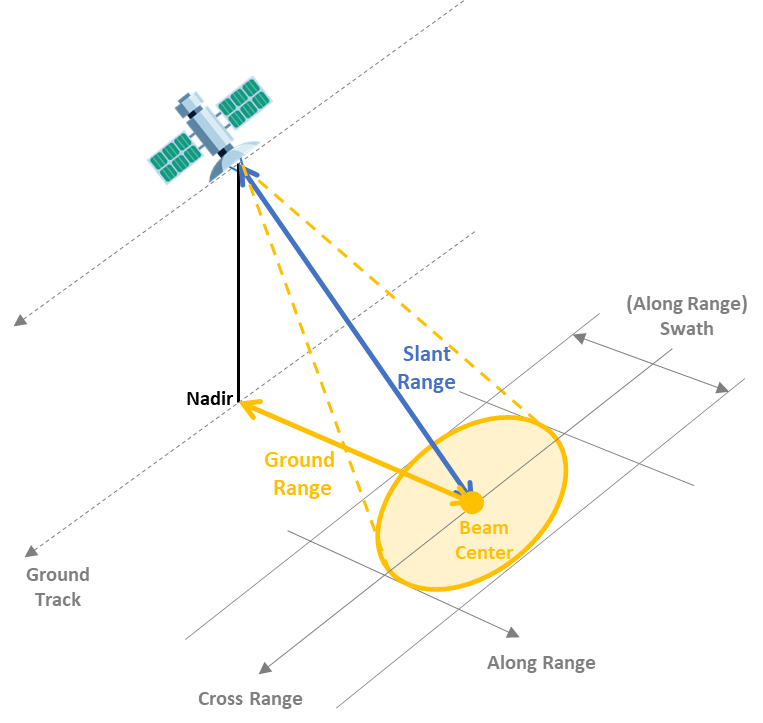```% Platform configuration v = 7e3; % Velocity (m/s) h = 565e3; % Altitude (m) % Radar signal configuration freq = 5.5e9; % Radar frequency (Hz) lambda = freq2wavelen(freq); % Wavelength (m) bw = 500e6; % Bandwidth (Hz) proctime = 0.8; % Processing time (s) % Antenna dimensions daz = 5.2; % Along azimuth (m) del = 1.1; % Along height (m)```

Real Antenna Beamwidth and Gain

Use the `ap2beamwidth` function to calculate the real antenna beamwidth.

`realAntBeamwidth = ap2beamwidth([daz del],lambda) % [Az El] (deg)`
```realAntBeamwidth = 2×1 0.6006 2.8391 ```

Use the `aperture2gain` function to calculate the antenna gain.

`antGain = aperture2gain(daz*del, lambda) % dBi`
```antGain = 43.8369 ```

Antenna Orientation

The depression angle is often used to define the antenna pointing direction.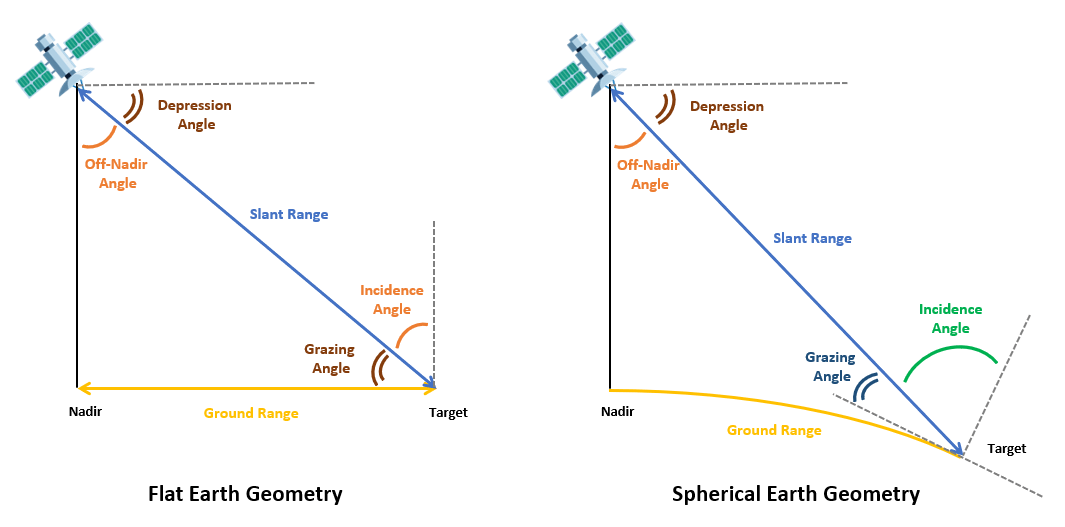Earth Curvature Effects

A typical assumption for many radar systems is that the Earth is flat so that the depression angle is the same as the grazing angle as shown in the figure.

```depang_flat = (45:85)'; grazang_flat = depang_flat; losrng = h./sind(depang_flat); % Line-of-sight range (m) ```

Use the `depressionang` and `grazingang` functions to calculate the depression and grazing angles respectively from the line-of-sight range. Using a spherical Earth model instead of the flat Earth model, observe that at ranges above 660 km the depression angle correction is greater than the half beamwidth, so it is critical to account for the Earth curvature in this scenario.

```Rearth = physconst('earthradius'); depang_sph = depressionang(h,losrng,'Curved',Rearth); grazang_sph = grazingang(h,losrng,'Curved',Rearth); plot(losrng/1e3,[depang_sph-depang_flat grazang_flat-grazang_sph],'Linewidth',1.5) grid on; yline(realAntBeamwidth(2)/2,'--') % Half-beam width (Elevation) xlabel('Line-of-sight Range (km)') ylabel('Angle Correction (deg)') legend('Depression angle','Grazing angle','Half Beamwidth (El)','Location','southeast') title(['Correction due to Earth Curvature—Radar at ',num2str(h/1e3),' km Altitude'])```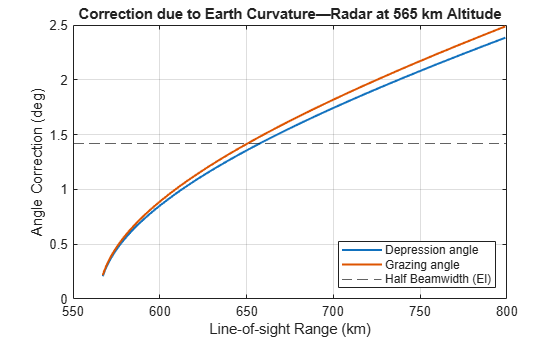Non-line-of-sight propagation

Radar energy is refracted by the atmosphere and bends towards the Earth, allowing the radar to see beyond the horizon. Use the `effearthradius` function to model the effect of tropospheric refraction using the average radius of curvature method. Since the effective Earth radius is equal to the actual Earth radius, you can conclude that the tropospheric refraction is negligible in this scenario. Ionospheric refraction is ignored in this scenario.

```tgtHeight = 0; % Smooth Earth NS = 313; % Reference atmosphere N-Units Re = effearthradius(min(losrng),h,tgtHeight,'SurfaceRefractivity',NS); % Effective Earth radius (m) Re/Rearth```
```ans = 1 ```

For the rest of this example, select a depression angle of 68.96 degrees, which corresponds to a grazing angle of 67 degrees and a slant range of 609.4 km.

`depang = depang_sph(24)`
```depang = 68.9629 ```
`grazang = grazang_sph(24)`
```grazang = 66.9953 ```
`slantrng = losrng(24)`
```slantrng = 6.0937e+05 ```

### Footprint and Resolution of Real and Synthetic Aperture Antennas

Next, calculate the antenna footprint using the `aperture2swath` function. The footprint is determined by the along range swath (or distance covered in the along range direction) and the cross-range swath (or distance covered in the cross-range direction).

```[rangeswath, crngswath] = aperture2swath(slantrng,lambda,[del daz],grazang); ['Real antenna range footprint: ', num2str(round(engunits(rangeswath),1)), ' km']```
```ans = 'Real antenna range footprint: 32.8 km' ```
`['Real antenna cross range footprint: ', num2str(round(engunits(crngswath),1)), ' km']`
```ans = 'Real antenna cross range footprint: 6.4 km' ```

Calculate the distance from the ground track (or nadir of the satellite) to the center of the radar beam on the ground. Notice that while the Earth curvature has a small effect on the footprint size, it shifts the beam center position by 9.5 km in the along range direction for a given slant range of 609.4 km.

```distgrndtrack_flat = h/tand(grazang_flat(24)); % Flat Earth Model ['Distance from nadir to center of the footprint (Flat Earth Model): ', num2str(round(engunits(distgrndtrack_flat),1)), ' km']```
```ans = 'Distance from nadir to center of the footprint (Flat Earth Model): 228.3 km' ```
```distgrndtrack = Re*deg2rad(depang-grazang); % Taking Earth curvature into account ['Distance from nadir to center of the footprint: ', num2str(round(engunits(distgrndtrack),1)), ' km']```
```ans = 'Distance from nadir to center of the footprint: 218.8 km' ```

Footprint Sensitivity to Frequency and Angle Variations

Analyze the antenna footprint sensitivity to frequency variations. Increasing the operating frequency of the radar reduces the antenna footprint.

```f = [freq/2 3/4*freq freq 4/3*freq]'; % Operating frequencies (Hz) within C-band lambdav = freq2wavelen(f); % Wavelengths (m) [rangeswathv,crngswathv] = aperture2swath(slantrng,lambdav,[del daz],grazang); clf; plotAntennaFootprintVsFreq(freq,f,rangeswathv,crngswathv);```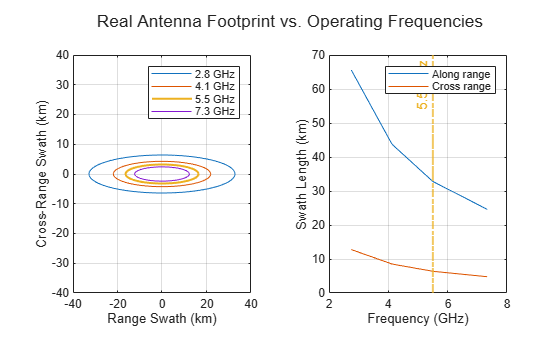Next, fix the operating frequency back to 5.5 GHz and analyze the antenna footprint sensitivity to grazing angle variations. Plot the distance from the center of the radar footprint to the satellite ground track (the ground range) as a function of look angles. As expected, the beam footprint and the beam center distance to nadir decrease when the grazing angle increases.

```grazv = grazang_sph(8:8:end); % Grazing angles (degrees) depangv = depang_sph(8:8:end); slantrngv = losrng(8:8:end); % Slant range to the ground rangeswathv = zeros(1,numel(grazv)); crngswathv = zeros(1,numel(grazv)); distgrndtrackv = Re*deg2rad(depangv-grazv); for i=1:numel(grazv) [rangeswathv(i),crngswathv(i)] = aperture2swath(slantrngv(i),lambda,[del daz],grazv(i)); end clf; plotAntennaFootprintVsGrazAng(grazv,distgrndtrackv,rangeswathv,crngswathv,grazang);```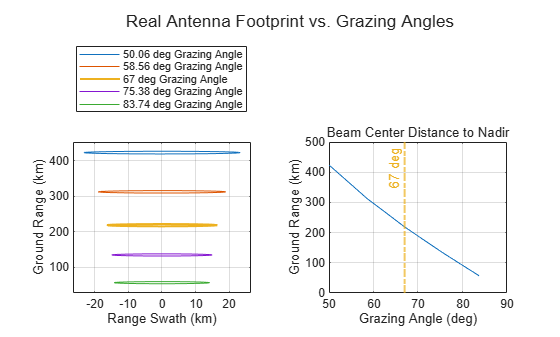Real Antenna Resolution

The ground range resolution is the distance below which two point targets cannot be separated in the final image. Use the `bw2rangeres` function to calculate the slant range resolution of the real antenna, which is determined by the signal bandwidth.

`slantrngres = bw2rangeres(bw)`
```slantrngres = 0.2998 ```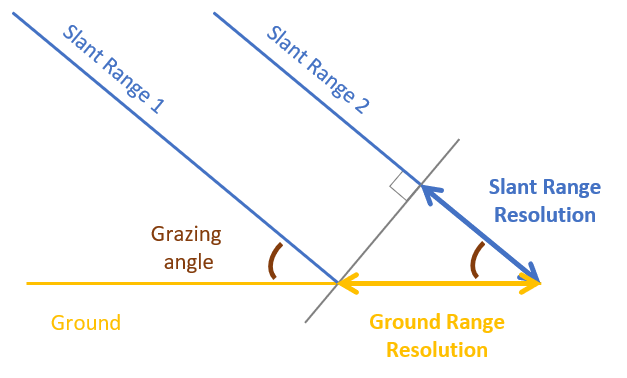Project the slant range resolution to the ground plane for a given grazing angle. The ground range resolution is always worse than the slant range resolution. The difference between the two increases as the grazing angle increases. Notice that the cross-range resolution is the same as the cross-range footprint since no signal processing is performed to enhance the resolution. This cross-range resolution is clearly insufficient.

```rngres = slant2grndrangeres(slantrngres,grazang); disp(['Real antenna ground range resolution: ', num2str(round(rngres,2)), ' m'])```
```Real antenna ground range resolution: 0.77 m ```
```crngres = crngswath; disp(['Real antenna cross-range resolution: ', num2str(round(engunits(crngres),1)), ' km'])```
```Real antenna cross-range resolution: 6.4 km ```

Next, analyze the ground range resolution sensitivity to variations of the grazing angle for a few signal bandwidths. The best range resolution is achieved with a high signal bandwidth and a low grazing angle. For a given bandwidth, the range resolution worsens as the grazing angle increases. At 500 MHz, the range resolution varies from 42 cm at a grazing angle of 45 degrees to 1.73 m at a grazing angle of 80 degrees.

```bwv = [100e6 200e6 500e6 1e9]; % Signal bandwidths rngresv = zeros(numel(grazang_sph),numel(bwv)); for j=1:numel(grazang_sph) slantrngresv = bw2rangeres(bwv); rngresv(j,:) = slant2grndrangeres(slantrngresv,grazang_sph(j)); end clf; l = semilogy(grazang_sph,rngresv); set(l(3),'LineWidth',2) grid on xline(grazang,'-.',{[num2str(round(grazang,2)),' deg']}); % Selected grazing angle xlabel('Grazing Angle (deg)') ylabel('Ground Range Resolution (m)') title('Real Antenna Range Resolution vs. Grazing Angles') legend('100 MHz BW', '200 MHz BW', '500 MHz BW', '1.0 GHz BW','Location','southeast')```Ideal vs Effective Resolution of the Synthetic Antenna

The previous section covered the range and cross-range resolution of a real aperture radar, the next step is to use the `sarlen` and `sarazres` functions to calculate the synthetic aperture length and its cross-range (or azimuth) resolution. Notice that the range resolution, which is dependent on the transmit signal bandwidth and the grazing angle, remains the same for a real aperture radar and a SAR.

```idealSyntheticApertureLength = sarlen(slantrng,lambda,daz); % Synthetic aperture length (m) azResolution = sarazres(lambda,slantrng,idealSyntheticApertureLength); % Cross-range (azimuth) resolution (m) integrationTime = sarinttime(idealSyntheticApertureLength,v); % Ideal integration time (s) Parameters = ["Synthetic Aperture Length";"Range Resolution";"Cross Range Resolution";"Integration Time"]; IdealSAR = [round(idealSyntheticApertureLength/1e3,1);rngres;round(azResolution,1);round(integrationTime*1e3,1)]; Units = ["km";"m";"m";"ms"]; idealSAR = table(Parameters,IdealSAR,Units)```
```idealSAR=4×3 table Parameters IdealSAR Units ___________________________ ________ _____ "Synthetic Aperture Length" 6.4 "km" "Range Resolution" 0.76711 "m" "Cross Range Resolution" 2.6 "m" "Integration Time" 1095.9 "ms" ```

The best cross-range resolution to use SAR in this scenario is 2.6 m. That is a considerable improvement compared to the 6.4 km cross-range resolution of the real antenna. However, to achieve this performance, pulses need to be integrated for over 1.1 s. The system you analyze in this example specifies an integration time of only 800 ms. This affects the effective cross-range resolution of the radar.

```effSyntheticApertureLength = sarlen(v,proctime); % Take processing time constraint into account effAzResolution = sarazres(lambda,slantrng,effSyntheticApertureLength); % Effective azimuth resolution (m) RealAntenna = [NaN; rngres; round(crngres); NaN]; EffectiveSAR = [round(effSyntheticApertureLength/1e3,1);rngres;round(effAzResolution,1);round(proctime*1e3,1)]; sar = table(Parameters,RealAntenna,IdealSAR,EffectiveSAR,Units)```
```sar=4×5 table Parameters RealAntenna IdealSAR EffectiveSAR Units ___________________________ ___________ ________ ____________ _____ "Synthetic Aperture Length" NaN 6.4 5.6 "km" "Range Resolution" 0.76711 0.76711 0.76711 "m" "Cross Range Resolution" 6388 2.6 3 "m" "Integration Time" NaN 1095.9 800 "ms" ```

By integrating pulses for only 800 ms, the synthetic aperture length is reduced by 800 m compared to the ideal case, and the cross-range resolution is reduced by 0.4 m to 3.0 m. Because data is processed in less than 1.11 s, the radar can keep the target within the beam footprint for the length of the computation.

Next, analyze the cross-range resolution sensitivity to frequency variations. The cross-range resolution improves at higher frequencies.

```azresv = sarazres(lambdav,slantrng,idealSyntheticApertureLength); effazresv = sarazres(lambdav,slantrng,effSyntheticApertureLength); plot([f f]/1e9,[azresv(:) effazresv(:)]) grid on legend('Ideal SAR','Effective SAR') xlabel('Frequency (GHz)') ylabel('Cross-range Resolution (m)') title('Cross Range Resolution vs. Operating Frequency')```### Range and Cross-Range Ambiguities in SAR Images

Coverage (swath length) and resolution cannot be chosen independently as they both impose constraints on the pulse repetition frequency (PRF). If the PRF is set too low, the radar suffers from grating lobes and Doppler (or cross-range) ambiguities. If the PRF is set too high, range measurements are ambiguous. The grazing angle also affects the PRF selection as it impacts the antenna footprint as seen in a previous section.

Upper and Lower PRF Bounds

Use the `sarprfbounds` function to determine the minimum and maximum PRF values for various range coverages (footprint) and cross-range resolutions given the satellite velocity and the grazing angle.

```desiredCRngRes = (0.5:0.2:5); % m desiredRngCov = (16e3:5e3:100e3); % m [prfminv, prfmaxv] = sarprfbounds(v,desiredCRngRes,desiredRngCov,grazang); clf plotPRFbounds(prfminv,desiredCRngRes,prfmaxv,desiredRngCov);```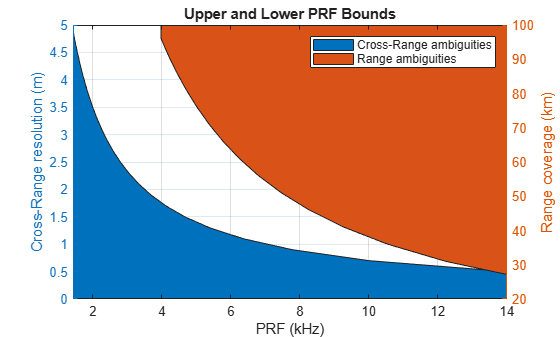PRF Selection to Avoid Ghost Images

The PRF is typically programmable and can be optimized for each mission. Use the `sarprf` function to calculate the PRF of the radar based on the satellite velocity and the real antenna dimension along azimuth. Specify a constant roll-off factor as a safety margin to prevent mainlobe returns from aliasing in the PRF interval.

`prf = sarprf(v,daz,'RollOff',1.5)`
```prf = 4.0385e+03 ```

The selected PRF is within the PRF bounds. The movement of the SAR platform within pulse repetition interval (PRI) is 1.73 m.

```t = 1/prf; distpri = sarlen(v,t) % Distance moved by satellite in one PRI```
```distpri = 1.7333 ```

Grating Lobes Analysis

Now model the synthetic array with the `phased.ula `System` `object using the effective aperture length and the element spacing.

```sarArray = phased.ULA('NumElements',ceil(effSyntheticApertureLength/distpri)+1,'ElementSpacing',distpri); distpri/lambda```
```ans = 31.7998 ```

Notice that the element spacing is over 31.8 times wavelength so the radar has grating lobes. Recall that the real antenna azimuth beamwidth is 0.6 degrees. Model the antenna response as a highly directional cosine pattern with the same beamwidth.

```realAnt = phased.CosineAntennaElement('FrequencyRange',[freq-bw/2 freq+bw/2],'CosinePower',26e3); realAntBeamwidth(1)```
```ans = 0.6006 ```
`b = beamwidth(realAnt,freq)`
```b = 0.6000 ```

Plot the pattern response of both the synthetic array and the real antenna to verify that the first grating lobe of the array is located in the null of the real antenna pattern, so the radar does not suffer negative impact from the grating lobes.

```clf plotResponse(sarArray,freq,physconst('LightSpeed'),'RespCut','Az','AzimuthAngles',(-3:0.005:3)); hold on plotResponse(realAnt,freq,'RespCut','Az','AzimuthAngles',(-3:0.005:3)); hold off legend('Synthetic array response','Real antenna response','Location','northwest') title('') % Annotations xl = xline(1.8,'-.',{'First grating lobe'}); xl.LabelVerticalAlignment = 'top'; xl.LabelHorizontalAlignment = 'left';```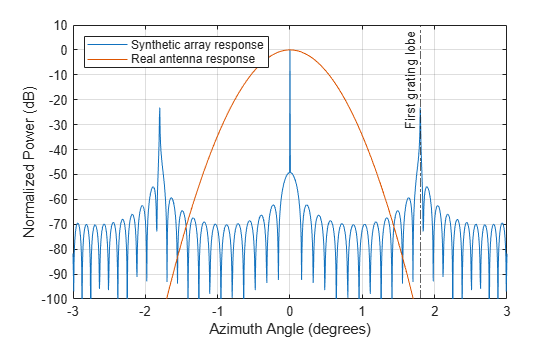### SAR Image SNR and Noise Equivalent Reflectivity

The next section examines different factors used in the SAR equation to calculate the image SNR. First, analyze the target (or surface) radar cross section (RCS) and the rain (or volume clutter) RCS.

Surface Roughness vs Frequency, Polarization and Grazing Angle

Use the `landreflectivity` function to calculate the reflectivity or the normalized radar cross section (NRCS) for a given grazing angle and operating frequency. The target RCS in the ground image plane is calculated using the `sarSurfaceRCS function` and taking the radar resolution into account. In general, the brightness of a SAR image area corresponds to the roughness of the surface so mountains appear brighter than flatland due to their higher RCS. Roughness is measured against wavelength so a surface appears rough at one frequency and smooth at another. Also, notice that the RCS increases as the grazing angle increases.

```fsub = [2.5e9 5.5e9 7.5e9]; % Hz grazv = 20:2:60; % degrees landtype = ["Mountains","Flatland"]; tgtrcsv = zeros(numel(grazv),numel(fsub),numel(landtype)); for k=1:numel(landtype) % Land types with different roughness for j=1:numel(fsub) % Frequencies for u =1:numel(grazv) % Grazing angles nrcsv = landreflectivity(landtype(k),grazv(u),fsub(j)); tgtrcsv(u,j,k) = sarSurfaceRCS(nrcsv,[slantrngres effAzResolution],grazv(u)); end end end plotTargetRCSvsGrazAng(grazv,tgtrcsv,landtype,fsub)```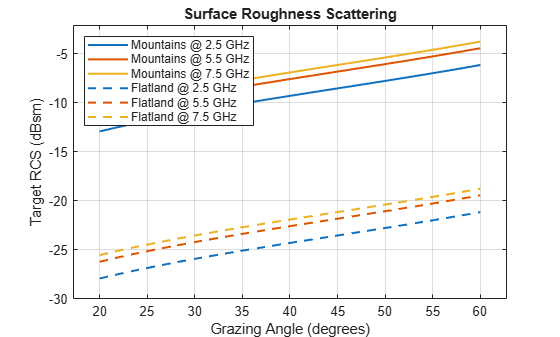RCS also depends on the polarization of the radar. Use the `seareflectivity` function to analyze the polarization effect on the sea surface reflectivity for different sea surface roughness (that is sea states). Note that there is much more variation in the sea RCS at low grazing angles and that the RCS for horizontally polarized signals is lower than vertically polarized signals for the same sea state for grazing angles below 60 degrees. This figure also shows that the difference between polarizations decreases as the grazing angle increases from 10 to 60 degrees.

```pol = ["H","V"]; seaStates = [1 3 5]; grazvpol = linspace(0.1,60,100); % Grazing angle (deg) tgtrcsv = zeros(numel(grazvpol),numel(pol),numel(seaStates)); for n=1:numel(seaStates) % Sea states for j=1:numel(pol) % Polarizations for u =1:numel(grazvpol) % Grazing angles nrcsv = seareflectivity(seaStates(n),grazvpol(u),freq,'Polarization',pol(j)); % Calculate normalized RCS tgtrcsv(u,j,n) = sarSurfaceRCS(nrcsv,[slantrngres effAzResolution],grazvpol(u)); end end end plotTargetRCSvsPol(grazvpol,tgtrcsv,seaStates,pol,freq)```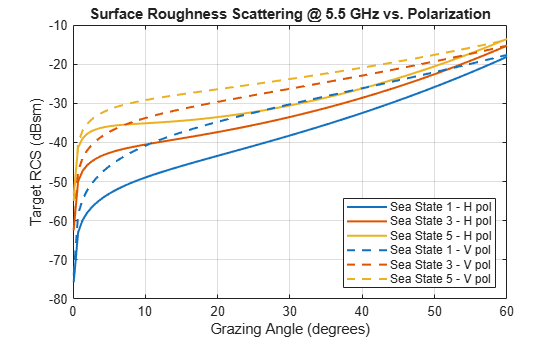Rain Reflectivity and Signal-to-Clutter Ratio (SCR)

Competing echoes from undesired sources such as rain can cause degradation in SAR images. Rain falling in the vicinity of a target scene clutters the image of that scene. Use the `rainreflectivity` function to analyze rain reflectivity under varying rain rates and polarizations. Observe that the rain reflectivity is higher for linear polarizations than circular polarization and that it increases with the rain rate.

```rainRate = [0.25 1 2 4 8 16]; pol = ["HH","HV","RCPRCP"]; rainrefl = zeros(numel(rainRate),numel(pol)); for i=1:numel(pol) rainrefl(:,i) = rainreflectivity(freq,rainRate,pol(i)); % 5.5 GHz operating frequency end plot(rainRate,pow2db(rainrefl),'LineWidth',1.5) grid on xlabel('Rain rate (mm/Hr)') ylabel('Rain Reflectivity (dB/m)') title('Polarization Effects on Rain Reflectivity') legend('HH Polarization','HV Polarization','Circular Polarization','Location','southeast');```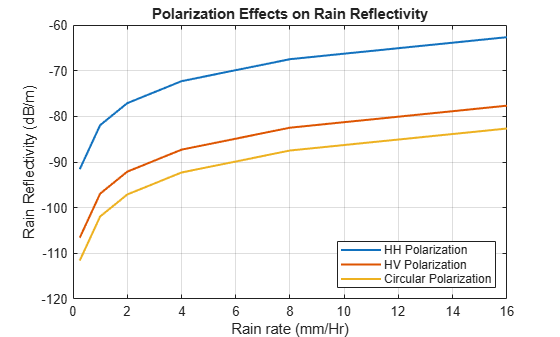Next, use the `clutterVolumeRCS` function to calculate the rain RCS, which is defined by the radar 3-D resolution cell (slant range, azimuth, and elevation resolution). Use the `rainscr` function to calculate the ratio of the signal energy from a resolution cell of the target scene to the energy from the rain returns processed into the same resolution cell of the target scene (or rain SCR). Verify that the signal-to-clutter ratio remains above 20 dB for all rain rates and for all targets including the ones with a weak RCS such as smooth land. Therefore, this example does not expect rain to be a limiting factor for this radar in this configuration.

```elbeamw = realAntBeamwidth(2); % Radar elevation beamwidth maxRainHeight = 4000; % m rhoe = rainelres(slantrng,elbeamw,grazang,maxRainHeight); % Elevation resolution res = [slantrngres effAzResolution rhoe]; rrcs = clutterVolumeRCS(rainrefl(:,1),res); % Rain RCS for HH polarization % The grazing angle of the geometry is slightly outside the default Barton % land reflectivity model, so temporarily turn off the reflectivity % warnings for the following calculation. warning('off','radar:radar:outsideValidityRegion'); % Calculate SCR landType = ["Wooded Hills","Farm","Smooth"]; scr = zeros(numel(rainRate),numel(landType)); tgtrcs = zeros(numel(landType),1); for j=1:numel(landType) nrcsv(j) = landreflectivity(landType(j),grazang,freq); % Calculate normalized RCS tgtrcs(j) = sarSurfaceRCS(nrcsv(j),[slantrngres effAzResolution],grazang); scr(:,j) = rainscr(lambda,rrcs,tgtrcs(j),proctime)'; % Signal to rain clutter ratio end % Turn reflectivity model warnings back on warning('on','radar:radar:outsideValidityRegion'); plotRainSCR(rainRate,rrcs,scr,landType)```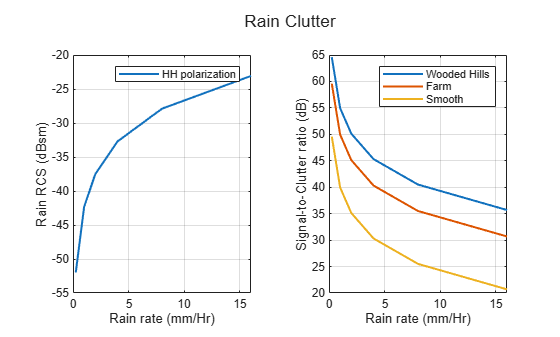SAR Equation

Estimate the signal-to-noise ratio (SNR) available at the radar receiver using the SNR form of the radar equation. First, model and estimate the different sources of gain and loss for the radar system and its environment.

Processing Gains

Use the `matchinggain` function to calculate the range processing gain due to the noise bandwidth reduction after the matched filter.

```d = 0.1; % 10 percent duty cycle pw = (1/prf)*d; % Effective pulse width (s) rnggain = matchinggain(pw,bw) % Range processing gain (dB)```
```rnggain = 40.9275 ```

Use the `sarazgain` function to calculate the azimuth processing gain due to the coherent integration of pulses.

`azgain = sarazgain(slantrng,lambda,v,effAzResolution,prf) % Azimuth processing gain (dB)`
```azgain = 35.0931 ```

Losses and Noise Factor

Use the `noisefigure` function to estimate the noise figure of the cascaded receiver stages. Assume seven stages with the following values:

• Stage 1 LNA: Noise Figure = 1.0 dB, Gain = 15.0

• Stage 2 RF Filter: Noise Figure = 0.5 dB, Gain = -0.5

• Stage 3 Mixer: Noise Figure = 5.0 dB, Gain = -7.0

• Stage 4 IF Filter: Noise Figure = 1.0 dB, Gain = -1.0

• Stage 5 IF Preamplifier: Noise Figure = 0.6 dB, Gain = 15.0

• Stage 6 IF Stages: Noise Figure = 1.0 dB, Gain = 20.0

• Stage 7 Phase Detectors: Noise Figure = 6.0 dB, Gain = -5.0

```nf = [1.0, 0.5, 5.0, 1.0, 0.6, 1.0, 6.0]; % dB g = [15.0, -0.5, -7.0, -1.0, 15.0, 20.0, -5.0]; % dB cnf = noisefigure(nf, g)```
```cnf = 1.5252 ```

Use the `radarpropfactor` function to calculate the one-way radar propagation factor over smooth land.

```[hgtsd, beta0, vegType] = landroughness('Smooth'); tgtheight = hgtsd; Re = effearthradius(slantrng,h,tgtheight); propf = radarpropfactor(slantrng,freq,h,tgtheight,'EffectiveEarthradius',Re,'TiltAngle',grazang,'ElevationBeamwidth',elbeamw,... 'SurfaceHeightStandardDeviation',hgtsd,'SurfaceSlope',beta0,'VegetationType',vegType)```
```propf = -5.3980e-05 ```

Use the `tropopl` function to calculate losses due to atmospheric gaseous absorption.

`atmoLoss = tropopl(slantrng,freq,tgtheight,grazang)`
```atmoLoss = 0.0439 ```

Use the `rainpl` function to calculate losses due to rain according to the ITU model. Alternatively, you can use the `cranerainpl` function to make calculations according to the Crane model.

`rainLoss = rainpl(slantrng,freq,rainRate(end),grazang)`
```rainLoss = 0.5389 ```

Use the `radareqsarsnr` function to calculate the image SNR with the SAR radar equation. Assume a 5 kW peak power. You can also specify additional losses and factors including the azimuth beam shape loss, window loss, transmission loss, and receive line loss. Estimate the beam shape loss with the `beamloss` function and use 5 dB for all other fixed losses combined. For this analysis, specify `landType` as "`Smooth`" to use the weakest land target. A minimum image SNR of 10 dB is usually regarded as sufficient to provide a good image quality, so at 35.9 dB for this target the system has ample margins.

```Lb = beamloss; customLoss = 5; % dB Pt = 5e3; % Peak power (W) sntemp = systemp(cnf); % Noise Temperature imgsnr = radareqsarsnr(slantrng,lambda,Pt,pw,rnggain,azgain,'Gain',antGain,'RCS',tgtrcs(3),... 'AtmosphericLoss',atmoLoss,'Loss',cnf+rainLoss,'PropagationFactor',propf,... 'Ts',sntemp,'CustomFactor',-Lb-customLoss)```
```imgsnr = 35.9554 ```

Noise Equivalent Reflectivity (NER or NEZ0)

Finally, use the `sarnoiserefl` function to calculate the NER of the radar and analyze its sensitivity to frequency variations. The NER is the smallest distributed clutter that can be seen in the presence of receiver noise for a given surface reflectivity. It is a measure of the sensitivity of the radar to spatially distributed noise. For the smooth terrain in this calculation, the NER is 62.6 dB at 5.5.GHz, and it increases with frequency.

```neq = sarnoiserefl(f,freq,imgsnr,nrcsv(3)); clf; plot(f/1e9,neq,'LineWidth',1.5) grid on xline(5.5,'--') xlabel('Frequency (GHz)') ylabel('Noise Equivalent Reflectivity (dB)') title(['Smooth Terrain—', num2str(round(effAzResolution,1)), ' m Resolution'])```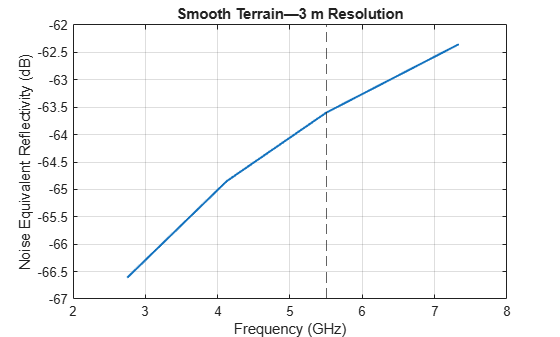### Summary

This example shows how to estimate performance parameters such as coverage, resolution and SNR for a spaceborne SAR system. First, you determine the upper and lower PRF bounds to avoid ghost images. Then you analyze target and rain clutter RCS for different polarizations and grazing angles. Then estimate processing gains and losses in the radar and its environment. Finally, you use the SAR equation to calculate the image SNR and the NER.

### References

1. Doerry, Armin Walter. “Performance Limits for Synthetic Aperture Radar.” Sandia National Laboratories, February 1, 2006.

2. O’Donnell, Robert. “Radar Systems Engineering .” IEEE Aerospace & Electronic Systems Society, and IEEE New Hampshire Section, 2013.

Supporting Functions

`slant2grndrangeres`

```function grndres = slant2grndrangeres(slres, grazang) % slant2grndrangeres Convert slant range resolution to ground range resolution grndres = slres./cosd(grazang); end```

`plotAntennaFootprintVsFreq`

```function t = plotAntennaFootprintVsFreq(freq,f,rangeswathv,crngswathv) t = tiledlayout(1,2); nexttile % Plot cross-range vs. range theta_grid = linspace(0,2*pi)'; semix = 0.5*rangeswathv/1e3; % km semiy = 0.5*crngswathv/1e3; % km l = plot(cos(theta_grid)*semix,sin(theta_grid)*semiy); set(l(3),'LineWidth',1.5) ylim(xlim) grid on xlabel('Range Swath (km)') ylabel('Cross-Range Swath (km)') legend([num2str(round(f(1)/1e9,1)) ' GHz'],[num2str(round(f(2)/1e9,1)) ' GHz'], ... [num2str(round(f(3)/1e9,1)) ' GHz'],[num2str(round(f(4)/1e9,1)) ' GHz']) nexttile % Plot Swath length vs. operating frequencies plot([f f]/1e9,[rangeswathv(:) crngswathv(:)]/1e3) xl = xline(freq/1e9,'-.',{[num2str(freq/1e9),' GHz']},'Color',l(3).Color); % Annotation xl.LabelVerticalAlignment = 'top'; xl.LabelHorizontalAlignment = 'left'; xl.LineWidth = 1.5; grid on xlabel('Frequency (GHz)') ylabel('Swath Length (km)') legend('Along range','Cross range') title(t,'Real Antenna Footprint vs. Operating Frequencies') end```

`plotAntennaFootprintVsGrazAng`

```function t = plotAntennaFootprintVsGrazAng(grazv,distgrndtrackv,rangeswathv,crngswathv,grazang) t = tiledlayout(1,2); nexttile % Plot footprint and beam center distance to ground track theta_grid = linspace(0,2*pi)'; semix = 0.5*rangeswathv/1e3; % km semiy = 0.5*crngswathv/1e3; % km l = plot(cos(theta_grid)*semix,1e-3*distgrndtrackv(:)'+sin(theta_grid)*semiy); set(l(3),'LineWidth',1.5) grid on xlabel('Range Swath (km)') ylabel('Ground Range (km)') legend([num2str(round(grazv(1),2)) ' deg Grazing Angle'], ... [num2str(round(grazv(2),2)) ' deg Grazing Angle'], ... [num2str(round(grazv(3),2)) ' deg Grazing Angle'], ... [num2str(round(grazv(4),2)) ' deg Grazing Angle'], ... [num2str(round(grazv(5),2)) ' deg Grazing Angle'], ... 'Location', "northoutside") axis padded nexttile % Plot beam center distance to ground track vs. grazing angles plot(grazv,distgrndtrackv/1e3) xl = xline(grazang,'-.',{[num2str(round(grazang,2)),' deg']},'Color',l(3).Color); % Annotation xl.LabelVerticalAlignment = 'top'; xl.LabelHorizontalAlignment = 'left'; xl.LineWidth = 1.5; grid on xlabel('Grazing Angle (deg)') ylabel('Ground Range (km)') subtitle('Beam Center Distance to Nadir') title(t,'Real Antenna Footprint vs. Grazing Angles') end ```

`plotPRFbounds`

```function plotPRFbounds(prfminv,desiredCRngRes,prfmaxv,desiredRngCov) yyaxis left fill([prfminv(1)/1e3;prfminv(:)/1e3;prfminv(end)/1e3],[0;desiredCRngRes(:);0],[0 0.4470 0.7410]) grid on xlabel('PRF (kHz)') ylabel('Cross-Range resolution (m)') yyaxis right fill([prfmaxv(1)/1e3;prfmaxv(:)/1e3;prfmaxv(end)/1e3],[100;desiredRngCov(:)/1e3;100],[0.8500 0.3250 0.0980]) xlim([prfminv(end)/1e3 prfminv(1)/1e3]) ylabel('Range coverage (km)') legend('Cross-Range ambiguities','Range ambiguities') title('Upper and Lower PRF Bounds') end ```

`plotTargetRCSvsGrazAng`

```function plotTargetRCSvsGrazAng(grazv,tgtrcsv,landtype,fsub) plot(grazv,pow2db(tgtrcsv(:,:,1)),'LineWidth',1.5) set(gca,'ColorOrderIndex',1) hold on plot(grazv,pow2db(tgtrcsv(:,:,2)),'--','LineWidth',1.5) hold off grid on axis padded xlabel('Grazing Angle (degrees)') ylabel('Target RCS (dBsm)') title('Surface Roughness Scattering') legend([char(landtype(1)) ' @ ' num2str(round(fsub(1)/1e9,1)) ' GHz'],... [char(landtype(1)) ' @ ' num2str(round(fsub(2)/1e9,1)) ' GHz'],[char(landtype(1)) ' @ ' num2str(round(fsub(3)/1e9,1)) ' GHz'],... [char(landtype(2)) ' @ ' num2str(round(fsub(1)/1e9,1)) ' GHz'],[char(landtype(2)) ' @ ' num2str(round(fsub(2)/1e9,1)) ' GHz'],... [char(landtype(2)) ' @ ' num2str(round(fsub(3)/1e9,1)) ' GHz'],'Location','northwest') end```

`plotTargetRCSvsPol`

```function plotTargetRCSvsPol(grazvpol,tgtrcsv,seaStates,pol,freq) plot(grazvpol,pow2db(squeeze(tgtrcsv(:,1,:))),'LineWidth',1.5) set(gca,'ColorOrderIndex',1) hold on plot(grazvpol,pow2db(squeeze(tgtrcsv(:,2,:))),'--','LineWidth',1.5) hold off grid on xlabel('Grazing Angle (degrees)') ylabel('Target RCS (dBsm)') title(['Surface Roughness Scattering @ ' num2str(round(freq/1e9,1)) ' GHz vs. Polarization']) legend(['Sea State ' num2str(seaStates(1)) ' - ' char(pol(1)) ' pol'],... ['Sea State ' num2str(seaStates(2)) ' - ' char(pol(1)) ' pol'],... ['Sea State ' num2str(seaStates(3)) ' - ' char(pol(1)) ' pol'],... ['Sea State ' num2str(seaStates(1)) ' - ' char(pol(2)) ' pol'],... ['Sea State ' num2str(seaStates(2)) ' - ' char(pol(2)) ' pol'],... ['Sea State ' num2str(seaStates(3)) ' - ' char(pol(2)) ' pol'],'Location','southeast') end ```

`plotRainSCR`

```function plotRainSCR(rainRate,rrcs,scr,landType) t = tiledlayout(1,2); nexttile % Plot rain RCS vs rain rate plot(rainRate,pow2db(rrcs),'LineWidth',1.5) grid on xlabel('Rain rate (mm/Hr)') ylabel('Rain RCS (dBsm)') legend('HH polarization') nexttile % Plot signal-to-clutter ratio (SCR) vs. rain rate plot(rainRate,scr,'LineWidth',1.5) grid on xlabel('Rain rate (mm/Hr)') ylabel('Signal-to-Clutter ratio (dB)') legend(landType(1),landType(2),landType(3)) title(t, 'Rain Clutter') end```